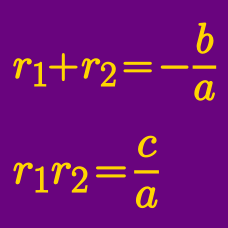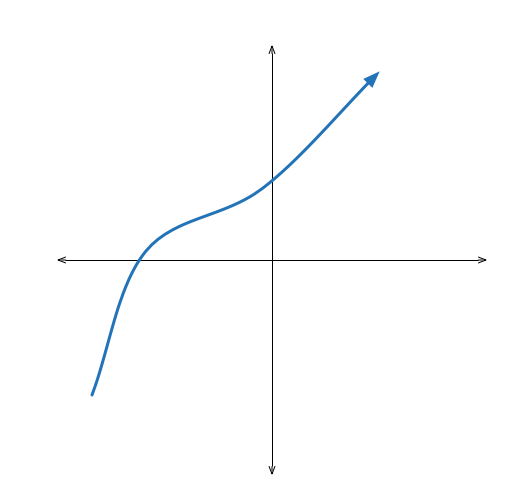Algebra

# Vieta's Formula: Level 3 Challenges

Jason and Thompson were solving the quadratic equation $$x^{2} + bx + c = 0$$.

Jason wrote down the wrong value of $$b,$$ and found the roots to be 6 and 1. Thompson wrote down the wrong value of $$c,$$ and found the roots to be $$-4$$ and $$-1.$$

What are the actual roots of the equation?

The non-zero roots of

$ax^2+bx+c=0$

are $$r$$ and $$s.$$ What are the roots of

$cx^2+bx+a = 0?$The roots of the polynomial equation $$x^3 + 2 x^2 + 3 x + 4 = 0$$ are $$\alpha, \beta,$$ and $$\gamma$$. What is the value of

$\frac{ \alpha + \beta} { \gamma} + \frac{ \beta + \gamma} { \alpha } + \frac{ \gamma + \alpha } { \beta } ?$

If $$\alpha$$, $$\beta$$, and $$\gamma$$ are the roots of $$x^3-x-1=0$$, compute:

$\frac{1-\alpha}{1+\alpha}+\frac{1-\beta}{1+\beta}+\frac{1-\gamma}{1+\gamma}$

$\large (x-7)(x-3)(x+5)(x+1)=1680$

Find the sum of all $$x$$ that satisfy the equation above.

×

Problem Loading...

Note Loading...

Set Loading...